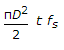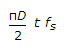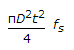# Civil Engineering - Theory of Structures - Discussion

Discussion Forum : Theory of Structures - Section 2 (Q.No. 21)
21.
For permissible shear stress fs, the torque transmitted by a thin tube of mean diameter D and wall thickness t, isπD2t fsExplanation:
No answer description is available. Let's discuss.
Discussion:
6 comments Page 1 of 1.

Jiban regmi said:   2 years ago
T = Fs*J/R.

where, J = π*(Do^4-Di^4)/32.
=π/32*(Do^2+Di^2)(Do+Di)(Do-Di).

= " * "* 2D *2t (where D=(Do+Di)/2, t=(Do-Di)/2).
=π*D*t/8 *[(Do-Di)^2+2*Do*Di].
=π*D*t/8*[ 4t^2 + 2D^2].
As the first term has a higher power of t , that can be neglected so;
=π*D^3*t/4.

Now Torque T=fs*J/R.
=π*D^2*t*fs/2.
(3)

Sri said:   3 years ago
@Aamir thickness is not in the formula.

Ashish Ganesh said:   4 years ago
What is the polar moment of inertia for the thin tube?

Aamir said:   4 years ago
T/J = fs/r.(torsion equation).
Find T.

Monika Verma said:   5 years ago
Anyone explain this.

Skm said:   6 years ago
How? Explain.